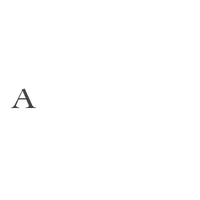Work, fun or play – our ON THE GO collection is for women who seamlessly excel at whatever the day brings them. Whether you are at work, dressing up for a date, partying with your besties or attending a formal event, this collection ensures you are always dressed to impress.

Rs. 120,000

Rs. 425,000

Rs. 585,000

Rs. 450,000

Rs. 180,000

Rs. 40,000

Rs. 205,000

Rs. 180,000

Rs. 90,000

Rs. 130,000

Rs. 80,000

Rs. 85,000

Rs. 140,000

Rs. 185,000

Rs. 90,000

Rs. 165,000

Rs. 142,000

Rs. 135,000

Rs. 205,000

Rs. 285,000

Rs. 115,000

Rs. 135,000

Rs. 255,000

Rs. 195,000

Rs. 175,000

Rs. 540,000

Rs. 170,000

Rs. 75,000

Rs. 40,000

Rs. 85,000

Rs. 95,000

Rs. 85,000

Rs. 85,000

Rs. 180,000

Rs. 140,000

Rs. 125,000

Rs. 135,000

Rs. 155,000

Rs. 168,000

Rs. 235,000

Rs. 60,000

Rs. 120,000

Rs. 1,300,000

Rs. 590,000

Rs. 590,000

Rs. 300,000

Rs. 665,000

Rs. 385,000

Rs. 185,000

Rs. 185,000

Rs. 385,000

Rs. 235,000

Rs. 225,000

Rs. 192,000

Rs. 198,000

Rs. 450,000

Rs. 185,000

Rs. 780,000

Rs. 780,000

Rs. 300,000

Rs. 888,000

Rs. 281,000

Rs. 225,000

Rs. 150,000

Rs. 275,000

Rs. 138,000

Rs. 250,000

Rs. 536,000

Rs. 188,000

Rs. 916,000

Rs. 1,032,000

Rs. 259,000

Rs. 166,000

Rs. 250,000

Rs. 250,000

Rs. 130,000

Rs. 300,000

Rs. 120,000

Rs. 95,000

Rs. 120,000

Rs. 810,000

Rs. 480,000

Rs. 218,000

Rs. 145,000

Rs. 110,000

Rs. 330,000